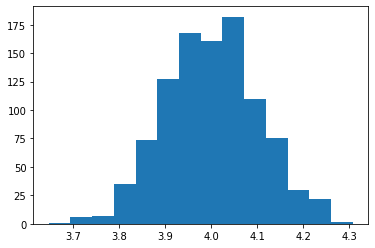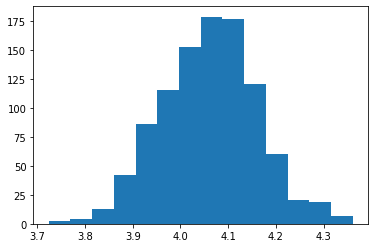In :
import pandas as pd
import numpy as np
import matplotlib
import matplotlib.pyplot as plt

from sklearn import tree
from sklearn.ensemble import BaggingClassifier, RandomForestClassifier

import graphviz


# Tree-Based Methods¶

## 8.1 Classification tree¶

This problem involves the OJ dataset (data/oj.csv)
Orange Juice Data

The data contains 1070 purchases where the customer either purchased Citrus Hill or Minute Maid Orange Juice. A number of characteristics of the customer and product are recorded.

A data frame with 1070 observations on the following 18 variables:

Purchase :A factor with levels CH and MM indicating whether the customer purchased Citrus Hill or Minute Maid Orange Juice
WeekofPurchase: Week of purchase
StoreID: Store ID
PriceCH: Price charged for CH
PriceMM: Price charged for MM
DiscCH: Discount offered for CH DiscMM: Discount offered for MM
SpecialCH: Indicator of special on CH
SpecialMM: Indicator of special on MM
LoyalCH: Customer brand loyalty for CH
SalePriceMM: Sale price for MM
SalePriceCH: Sale price for CH
PriceDiff: Sale price of MM less sale price of CH
Store7: A factor with levels No and Yes indicating whether the sale is at Store 7
PctDiscMM: Percentage discount for MM
PctDiscCH: Percentage discount for CH
ListPriceDiff: List price of MM less list price of CH
STORE: Which of 5 possible stores the sale occured at

### a)¶

Create a training set containing a random sample of 800 observations, and a test set containing the remaining observations.

In :
np.random.seed(1)

# sampling indices for training
trainIndex = np.random.choice(OJ.shape, size=800, replace=False)
train = OJ.iloc[trainIndex]                     # training set
test = OJ.iloc[~OJ.index.isin(trainIndex)]      # test set

In :
OJ.info()

<class 'pandas.core.frame.DataFrame'>
RangeIndex: 1070 entries, 0 to 1069
Data columns (total 18 columns):
Purchase          1070 non-null object
WeekofPurchase    1070 non-null int64
StoreID           1070 non-null int64
PriceCH           1070 non-null float64
PriceMM           1070 non-null float64
DiscCH            1070 non-null float64
DiscMM            1070 non-null float64
SpecialCH         1070 non-null int64
SpecialMM         1070 non-null int64
LoyalCH           1070 non-null float64
SalePriceMM       1070 non-null float64
SalePriceCH       1070 non-null float64
PriceDiff         1070 non-null float64
Store7            1070 non-null object
PctDiscMM         1070 non-null float64
PctDiscCH         1070 non-null float64
ListPriceDiff     1070 non-null float64
STORE             1070 non-null int64
dtypes: float64(11), int64(5), object(2)
memory usage: 150.6+ KB


### b)¶

Learn a classification tree from the training data using the function sklearn.tree.DecisionTreeClassifier(), with Purchase as the output and the other variables as inputs. Don't forget to handle qulitative variables correctly. To avoid severe overfit, you have to add some constraints to the tree, using, e.g., a maximum depth of 2 (max_depth=2).

In :
X_train = train.drop(columns=['Purchase'])
# Need to transform the qualitative variables to dummy variables
X_train = pd.get_dummies(X_train, columns=['Store7'])
y_train = train['Purchase']
model = tree.DecisionTreeClassifier(max_depth=2)
model.fit(X=X_train, y=y_train)

Out:
DecisionTreeClassifier(class_weight=None, criterion='gini', max_depth=2,
max_features=None, max_leaf_nodes=None,
min_impurity_decrease=0.0, min_impurity_split=None,
min_samples_leaf=1, min_samples_split=2,
min_weight_fraction_leaf=0.0, presort=False,
random_state=None, splitter='best')

### c)¶

Use sklearn.tree.export_graphviz()and the Python module graphviz to visualize the tree and interpret the result. How many terminal nodes does the tree have? Pick one of the terminal nodes, and interpret the information displayed. Type OJ.info() to get information about all input variables in the data set.

In :
dot_data = tree.export_graphviz(model, out_file=None, feature_names = X_train.columns,
class_names = model.classes_, filled=True, rounded=True,
leaves_parallel=True, proportion=True)
graph = graphviz.Source(dot_data)
graph

Out:

### d)¶

Predict the response on the test data, and produce a confusion matrix comparing the test labels to the predicted test labels. What is the test error rate?

In :
X_test = test.drop(columns=['Purchase'])
X_test = pd.get_dummies(X_test, columns=['Store7'])
y_test = test['Purchase']
y_predict = model.predict(X_test)
print('Accuracy rate is %.2f' % np.mean(y_predict == y_test))
pd.crosstab(y_predict, y_test)

Accuracy rate is 0.80

Out:
Purchase CH MM
row_0
CH 142 24
MM 30 74

### e)¶

Explore the different parameters you can pass to the tree command, such as splitter, min_samples_split, min_samples_leaf, min_impurity_split etc.

## 8.2 Random Forest¶

In this exercise we will use the email-spam data that has been presented in a couple of lectures. Go to the URL http://archive.ics.uci.edu/ml/datasets/Spambase for more information about the data.

### a)¶

Load the dataset data/email.csv

In :
# url = 'data/email.csv'
url = 'https://uu-sml.github.io/course-sml-public/data/email.csv'


### (b)¶

Create a training set containing a random sample of $75\%$ of the observations, and a test set containing the remaining observations.

In :
np.random.seed(1)
trainIndex = np.random.choice(email.shape,size=int(len(email)*.75),replace=False)
train = email.iloc[trainIndex]                     # training set
test = email.iloc[~email.index.isin(trainIndex)]      # test set


### (c)¶

Fit a classification tree with Class as output. Compute the test error.

In :
X_train = train.copy().drop(columns=['Class'])
y_train = train['Class']
X_test = test.copy().drop(columns=['Class'])
y_test = test['Class']

In :
model = tree.DecisionTreeClassifier(max_leaf_nodes=5)
model.fit(X=X_train, y=y_train)
y_predict = model.predict(X_test)
print('Test error rate is %.3f' % np.mean(y_predict != y_test))

Test error rate is 0.111


### (d)¶

Use the bagging approach to learn a classifier sklearn.ensemble.BaggingClassifier(). What test error do you get?

In :
model = BaggingClassifier()
model.fit(X_train, y_train)
error = np.mean(model.predict(X_test) != y_test)
print('Test error: %.3f' % error)

Test error: 0.063


### (e)¶

Learn a random forest classifier using the function sklearn.ensemble.RandomForestClassifier(). What test error do you get?

In :
model = RandomForestClassifier(n_estimators=100)
model.fit(X_train, y_train)
error = np.mean(model.predict(X_test) != y_test)
print('Test error: %.3f' % error)

Test error: 0.036


## 8.3 Bootstrap¶

The bootstrap method has been introduced to reduce the variance in decision trees. However, the bootstrap method is widely applicable in other context as well, for example to estimate the variance in a parameter (cf. bias-variance tradeoff).

### (a)¶

Generate $n = 100$ samples $\{y_i\}_{i=1}^n$ from $\mathcal{N}(4, 1^2)$.

In :
np.random.seed(0)
n = 100
y = np.random.normal(4, 1, n)


### (b)¶

We want to lean a model $y = \theta_0 + \epsilon$ (with $\mathbb{E}[\epsilon]=0$) from the data $\{y_i\}_{n=1}^n$. Estimate $\theta_0$ with least squares, i.e. $\hat{\theta}_0 = \frac{1}{n}\sum_{i=1}^n y_i$.

In :
theta_0 = np.mean(y)
theta_0

Out:
4.059808015534485

### (c)¶

To estimate the variance in $\hat{\theta}_0$, $\operatorname{Var}[\hat{\theta}_0]$ (a possible ‘quality measure’ of an estimator), repeat (a)-(b) $1000$ times to get $1000$ estimates of $\theta_0$, which we call $\hat{\theta}_0^1, ..., \hat{\theta}_0^{1000}$. Plot a histogram over the estimates $\hat{\theta}_0^1, ..., \hat{\theta}_0^{1000}$.

In :
theta = []
for i in range(1000):
theta.append(np.mean(np.random.normal(4, 1, n)))

plt.hist(theta, bins=14)
plt.show()In practice we only have access to $\{y_i\}_{n=1}^n$ $(n = 100)$ and cannot estimate $\hat{\theta}_0^1, ..., \hat{\theta}_0^{1000}$ by generating new data. However, with the bootstrap method we can obtain ‘new’ data sets by sampling from the original data set $\{y_i\}_{n=1}^n$.

### (d)¶

Sample $n$ indices $\{i_1, i_2, ... , i_n\}$ with replacement from the set $\{0, 1, ... , n-1\}$ using the function numpy.random.choice(). Note that some indices will appear multiple times and some will not appear at all.

In :
indices = np.random.choice(np.arange(100), size=n, replace=True)
indices

Out:
array([77, 91, 78, 64, 51, 12, 89,  8, 33, 70, 35, 74, 84, 91, 74, 78, 75,
80, 40, 35, 97, 56, 16, 38, 86, 80, 98, 63, 11, 53, 32, 30, 75, 44,
7, 41, 97, 77, 60, 36, 63, 27, 61, 57, 66, 66, 48,  2, 25, 93, 56,
78, 33, 71, 64, 14, 68, 25, 28, 26, 76, 22, 30, 23, 51, 16, 24, 27,
74, 66, 80, 91, 43, 37,  6, 97, 91,  4, 83, 94, 62, 71,  1, 28, 64,
45, 19,  0, 72, 11, 86, 59, 90, 41, 13, 78, 25, 30, 48, 28])

### (e)¶

Generate a ‘new’ data set $\{y_j\}_{j=1}^n$ based on the indices generated in (d) and estimate $\hat{\mu}$ from this data set.

In :
y_new = y[indices]


### (f)¶

Repeat (d)-(e) $1000$ times to get $1000$ estimates of $\hat{\mu}$, which we call $\hat{\mu}_1^*, ..., \hat{\mu}_{1000}^*$. Plot a histogram over the estimates $\hat{\mu}_1^*, ..., \hat{\mu}_{1000}^*$ and compare with the estimate achieved in (c).

In :
mu = []
for i in range(1000):
y_new = np.random.choice(y, size=n, replace=True)
mu.append(np.mean(y_new))

plt.hist(mu, bins=14)
plt.show()

# The distribution of the estimates very similar to the first histogram,
# but centered around $4.04$ which was the mean of this data set. Consequently,
# we got a fairly accurate estimate of the uncertainity of the estimate without
# generating new data. For a linear estimator like this one, the uncertainity
# can be computed analytically (try to do that!), but in many nonlinear cases,
# this is not tractable or even possible. Then, the bootstrap method is very
# powerful.